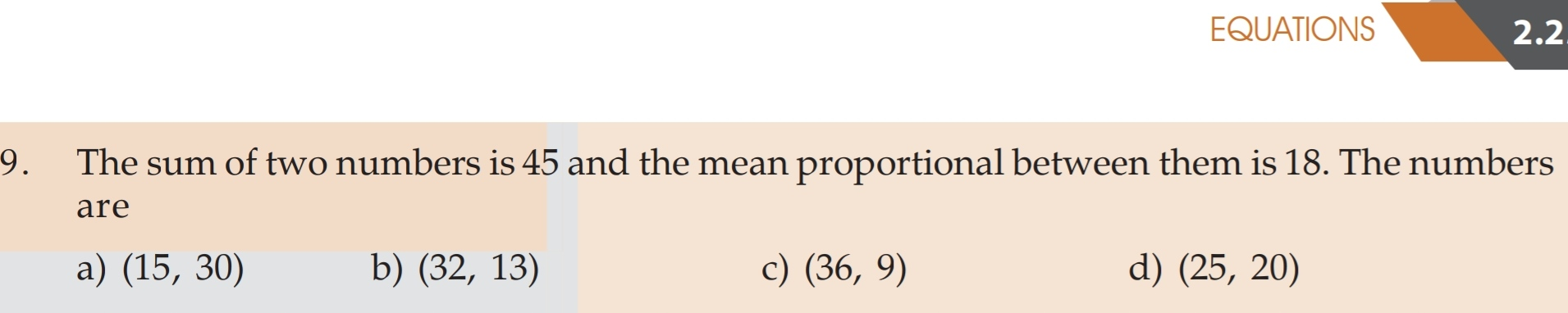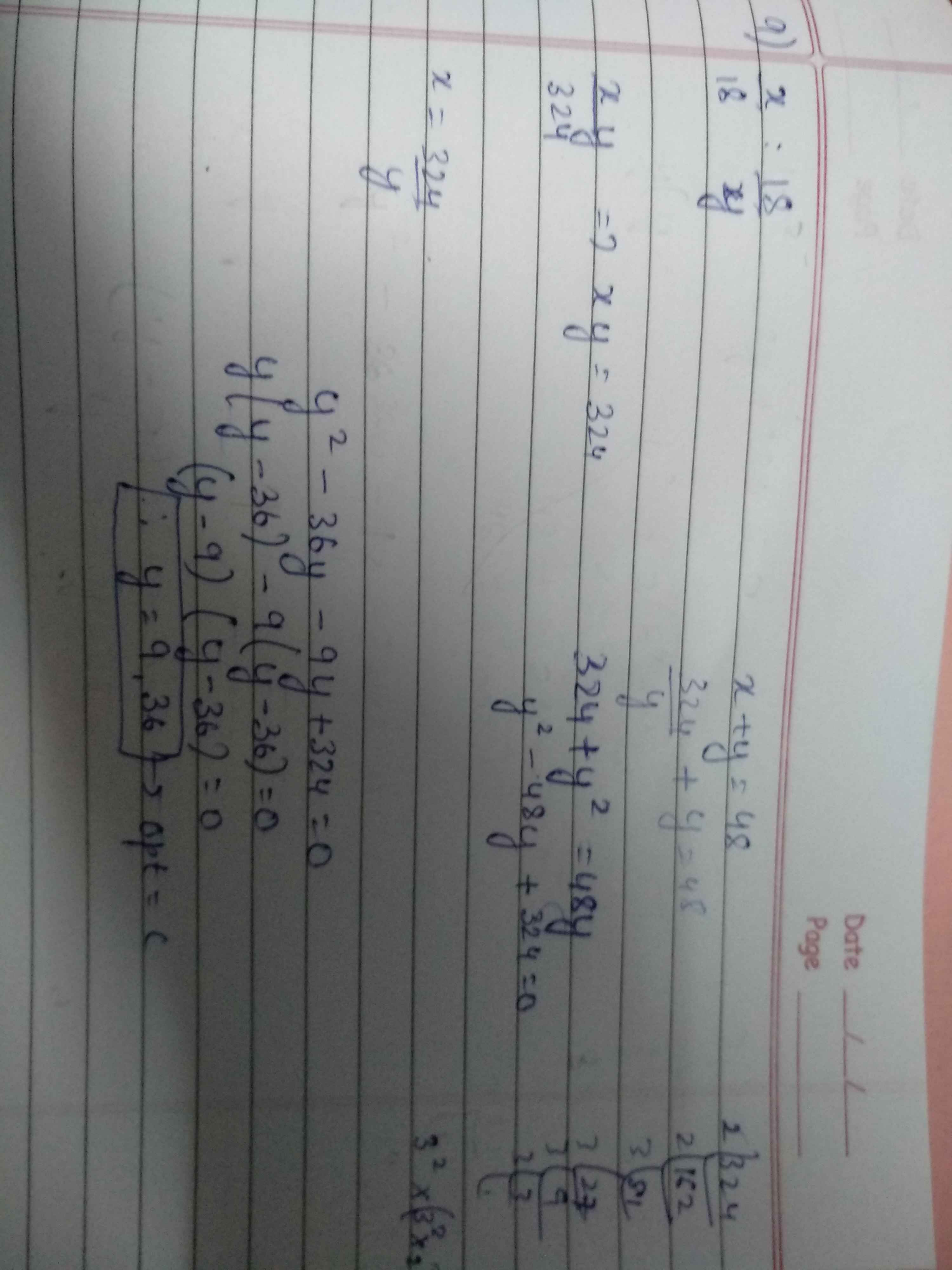Forums

# Equations

Sir/ma'amHow to solve when mean proportion is givenSoumya A

CA Inter

20K+

03-Aug-20

106

Sir/ma'am I have done it in this way is it correct or notSoumya A

CA Inter

20K+

04-Aug-20

Let numbers be x& y x+y = 45 and square root of xy = 18 and then substitute value of y i.e. y=45-x in the second equation i.e. xy=18^2=324. Then u will get 36 & 9sudha reddy

Moderator

04-Aug-20

Sales